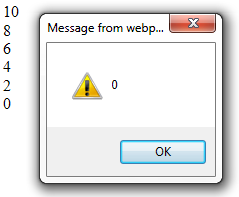Support us .Net Basics C# SQL ASP.NET Aarvi MVC Slides C# Programs Subscribe Download

### JavaScript array push and pop methods

Suggested Videos
Part 17 - do while loop in JavaScript
Part 18 - For loop in JavaScript
Part 19 - Arrays in javascript

In this video we will discuss push() and pop() methods in JavaScript. Along the way we will also discuss shift() and unshift() methods. Let us understand these methods with examples.

In the example below, we are populating myArray using a for loop and the array index. Subsequently we are using another for loop to retrieve the elements from the array. Finally we are displaying the length of the array using JavaScript alert.
var myArray = [];

for (var i = 0; i <= 5; i++)
{
myArray[i] = i * 2;
}

for (var i = 0; i <= 5; i++)
{
document.write(myArray[i] + "<br/>");
}

Output :Please note : Retrieving array elements using the array index, will not change the length of the array.

JavaScript push() method
This method adds new items to the end of the array. This method also changes the length of the array.

JavaScript pop() method
This method removes the last element of an array, and returns that element. This method changes the length of an array.

Example : In the exampe below, we are using push() method to populate the array and pop() method to retrieve elements from the array. Notice that push() and pop() methods change the length property of the array.
var myArray = [];

for (var i = 0; i <= 5; i++)
{
myArray.push(i * 2);
}

for (var i = 0; i <= 5; i++)
{
document.write(myArray.pop() + "<br/>");
}

Output :JavaScript unshift() Method
push() method adds new items to the end of the array. To add new items to the beginning of an array, then use unshift() method. Just like push() method, unshift() method also changes the length of an array

Example :
var myArray = [2, 3];

// Adds element 4 after element 3
myArray.push(4);

// Adds element 1 before element 2
myArray.unshift(1);

document.write("Array elements = " + myArray + "<br/>");
document.write("Array Length = " + myArray.length);

Output :JavaScript shift() Method
pop() method removes the last element of an array, and returns that element. shift() method removes the first item of an array, and returns that item. Just like pop() method, shift() method also changes the length of an array.

Example :
var myArray = [1, 2, 3, 4, 5];

// removes the last element i.e 5 from the array
var lastElement = myArray.pop();
document.write("Last element = " + lastElement + "<br/>");

// removes the first element i.e 1 from the array
var firstElement = myArray.shift();
document.write("First element = " + firstElement + "<br/><br/>");

document.write("Array elements = " + myArray + "<br/>");
document.write("Array Length = " + myArray.length);

Output :